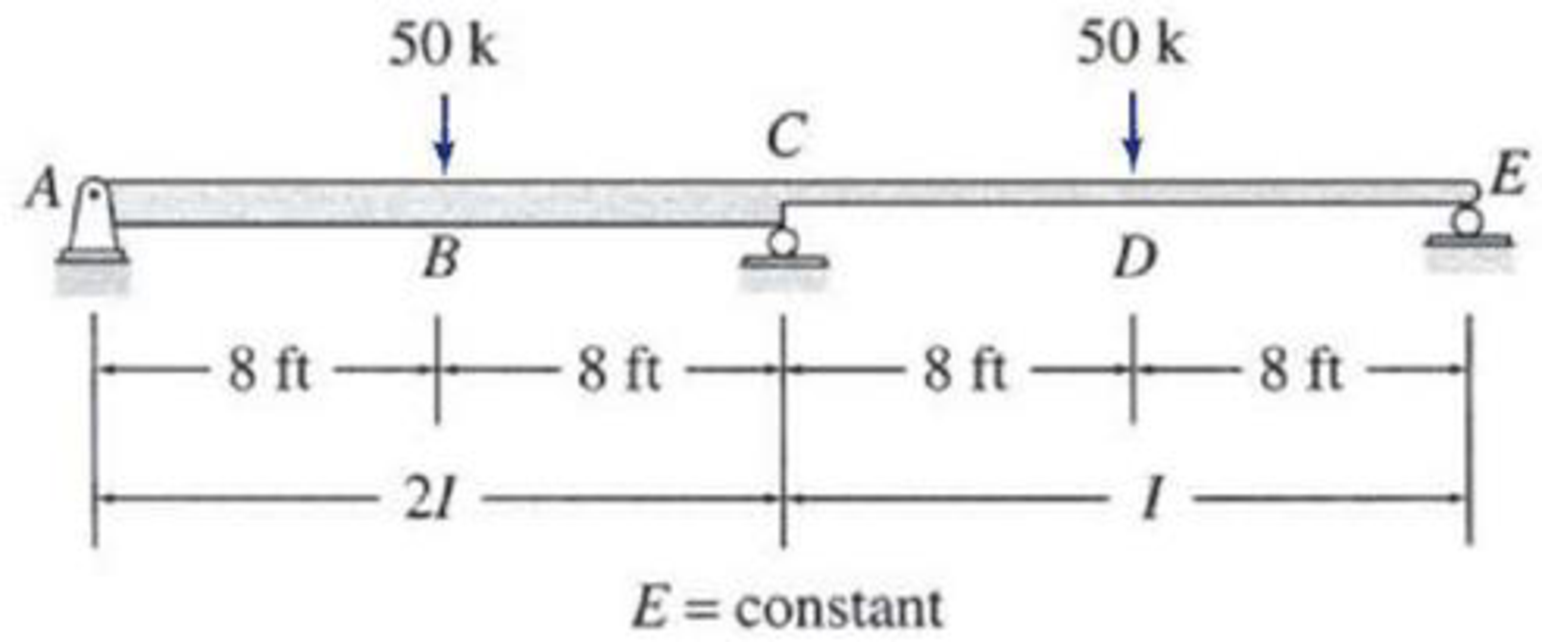# 13.9 through 13.12 Determine the reactions and draw the shear and bending moment diagrams for the beams shown in Figs. P13.9–P13.12 using the method of consistent deformations. Select the reaction at the interior support to be the redundant. FIG. P13.11, P13.32

#### Solutions

Chapter
Section
Chapter 13, Problem 11P
Textbook Problem
164 views

## 13.9 through 13.12 Determine the reactions and draw the shear and bending moment diagrams for the beams shown in Figs. P13.9–P13.12 using the method of consistent deformations. Select the reaction at the interior support to be the redundant.FIG. P13.11, P13.32

To determine

Calculate the support reactions for the given structure using method of consistent deformation.

Sketch the shear and bending moment diagrams for the given structure.

### Explanation of Solution

Given information:

The structure is given in the Figure.

Apply the sign conventions for calculating reactions, forces and moments using the three equations of equilibrium as shown below.

• For summation of forces along x-direction is equal to zero (Fx=0), consider the forces acting towards right side as positive (+) and the forces acting towards left side as negative ().
• For summation of forces along y-direction is equal to zero (Fy=0), consider the upward force as positive (+) and the downward force as negative ().
• For summation of moment about a point is equal to zero (Matapoint=0), consider the clockwise moment as positive when moment taken from left and the counter clockwise moment as positive when moment taken from right.

Calculation:

Sketch the free body diagram of the beam as shown in Figure 1.

Refer Figure 1.

Find the degree of indeterminacy of the structure:

Degree of determinacy of the beam is equal to the number of unknown reactions minus the number of equilibrium equations.

The beam is supported by 4 support reactions (r) and the number of equilibrium equations (e) is 3.

i=re=43=1

Therefore, the degree of indeterminacy of the beam is i=2.

Select the vertical reaction Cy at the supports C as redundant.

Release the support C and consider the downward deflection due to external load at C as ΔCO

Sketch the loading diagram with deflected shape as shown in Figure 2.

Refer Figure 2.

Find the vertical reaction at A and C.

AyO=EyO=50k

Find the moment at A. (hinged support):

MAO=0

The moment of inertia of portion AB is doubled. The moment of the portion AC is the half of the moment of the portion CE. Divide the moment by 2 in the portion AC.

Find the moment at B from A. (I=2I).

MBO=(AyO×8)2

Substitute 50 k for AyO.

MBO=(50×8)2=200k-ft

Find the moment at C from A. (I=2I).

MCO=(AyO×16)50(8)2

Substitute 50 k for AyO.

MCO=(50×16)50(8)2=200k-ft

Find the moment at E. (roller support):

MEO=0

Find the moment at D from E.

MDO=EyO(8)

Substitute 50 k for EyO.

MDO=50(8)=400k-ft

Find the moment at C from E.

MCO=EyO(16)50(8)

Substitute 50 k for EyO.

MCO=50(16)50(8)=400k-ft

Sketch the MEI diagram using moment values as shown in Figure 3.

Refer Figure 3.

Find the vertical reaction at A in the MEI diagram.

Summation of moment at E is zero.

[AyEI(16)+12(8)(200EI)(83+24)+(8)(200EI)(82+16)+(8)(400EI)(82+8)+12(8)(400EI)(23×8)]=032AyEI+1EI(21,333.33+32,000+38,400+8,533.33)=032AyEI=1,00,266.66EIAyEI=3,133.33EI

Find the downward deflection at C.

The moment at C in the MEI diagram is the equal to the downward deflection at point C.

ΔCO=AyEI(16)+12(8)(200EI)(83+8)+(8)(200EI)(82)=(3,133.33EI)(16)+8,533.33EI+6,400EI=35,200EI

Find the upward deflection at B.

### Still sussing out bartleby?

Check out a sample textbook solution.

See a sample solution

#### The Solution to Your Study Problems

Bartleby provides explanations to thousands of textbook problems written by our experts, many with advanced degrees!

Get Started

Find more solutions based on key concepts
List the materials used in the fabrication of compact fluorescent lights.

Engineering Fundamentals: An Introduction to Engineering (MindTap Course List)

Why do app developers opt for web delivery? (161)

Enhanced Discovering Computers 2017 (Shelly Cashman Series) (MindTap Course List)

What is logical independence?

Database Systems: Design, Implementation, & Management

What does SDS stand for, and what is the purpose of SDSs?

Precision Machining Technology (MindTap Course List)

What is JDBC, and what is it used for?

Database Systems: Design, Implementation, & Management

What is a project plan? List what a project plan can accomplish.

Principles of Information Security (MindTap Course List)

Write the script for a one-minute explanation of basic project management concepts.

Systems Analysis and Design (Shelly Cashman Series) (MindTap Course List)

Why is it important to strike the arc only in the weld joint?

Welding: Principles and Applications (MindTap Course List)

If your motherboard supports ECC DDR3 memory, can you substitute non-ECC DDR3 memory?

A+ Guide to Hardware (Standalone Book) (MindTap Course List)